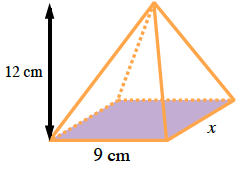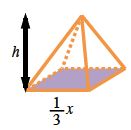Home > CCG > Chapter 11 > Lesson 11.2.1 > Problem11-86

11-86.

On your paper, draw a diagram of a square-based pyramid if the side length of the base is $9$ cm and the height of the pyramid is $12$ cm.

1. Find the volume of the pyramid.

The formula to find the volume of a pyramid is $\frac{1}{3}(\text{base})(\text{height})$

Calculate area of base:

$(9)(9) = 81\text{ cm}^2$

Use formula to calculate volume (a):

$\frac{1}{3}(12)(81) = \frac{1}{3}(972) = 324\: \text{units}^3$1. If a smaller pyramid is similar to the pyramid in part (a), but has a linear scale factor of $\frac { 1 } { 3 }$, find its volume.

Find the volume of the smaller pyramid in one of two ways:
Calculating from the dimensions or
Using the ratio of volumes.

Since the linear scale factor is $\frac{1}{3}$ ,the ratio of the volume is $(\frac{1}{3})^3$, $\frac{1}{27}$

$(324)\left(\frac{1}{27}\right) = 12 \text{ cm}^3$